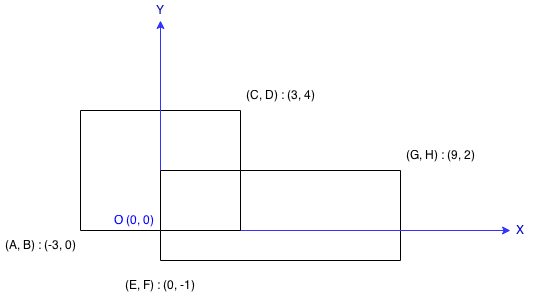### LeetCode• ㊗️
• 大家
• offer
• 多多！

## Problem

Find the total area covered by two rectilinear rectangles in a 2D plane.

Each rectangle is defined by its bottom left corner and top right corner as shown in the figure.Example:

Input: A = -3, B = 0, C = 3, D = 4, E = 0, F = -1, G = 9, H = 2
Output: 45


Note:

Assume that the total area is never beyond the maximum possible value of int.

## Code

class Solution {
public int computeArea(int A, int B, int C, int D, int E, int F, int G, int H) {
int res = 0;
int area1 = (C - A) * (D - B);
int area2 = (G - E) * (H - F);

int overlap = 0;

// 大左，小右
int leftMax = Math.max(A, E);
int rightMin = Math.min(C, G);
// 小上，大下
int topMin = Math.min(D, H);
int bottomMax = Math.max(B, F);

if(leftMax < rightMin && topMin > bottomMax){
overlap = Math.abs(rightMin - leftMax) * Math.abs(topMin - bottomMax);
}

return area1 + area2 - overlap;
}
}